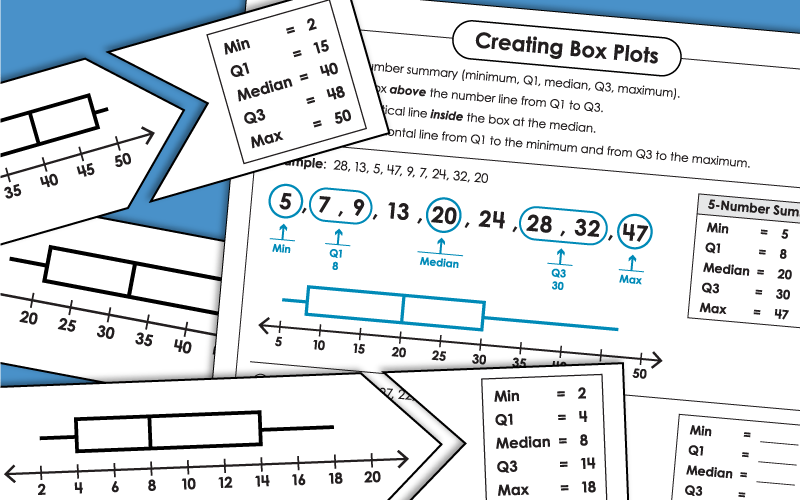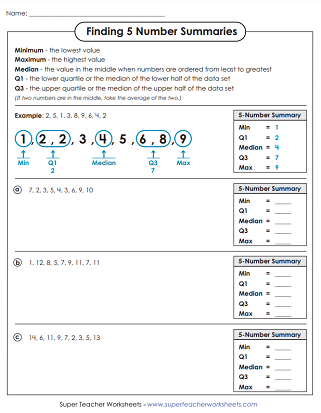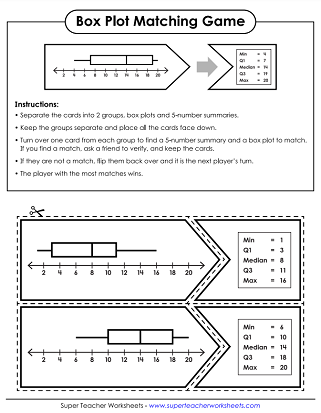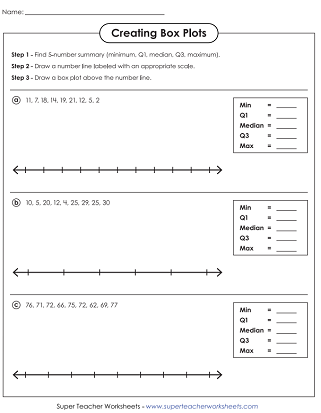# Box and Whisker Plots

Box plots (also known as box and whisker plots) are used in statistics and data analysis. They are used to show distribution of data based on a five number summary (minimum, first quartile Q1, median Q2, third quartile Q3, and maximum).## Level: Basic

Students are given sets of numbers. They'll need to find the minimum, maximum, median, Q1, and Q3. This basic level worksheets includes whole numbers 0-20.
Students are given the Q1, Q3, minimum, maximum, and mean. They use these numbers, along with a number line, to make a simple box and whisker plot.
Complete a 5-NS, create a BP, and answer the multiple choice questions.

## Level: Intermediate

Students are given five sets of 9 numbers. For each set, they are to find the median, Q1, Q3, minimum, and maximum. This intermediate-level worksheet has numbers between 0 and 100.
Use the numbers given to create a box plot with whiskers. This version has 2-digit numbers. Numbers range from 0 to 100.
These box plot problems have real word scenarios. Read each and draw a BP. Number range 0-100.
Cut out the puzzle pieces. Match each box plot with the correct 5-number summary. Numbers range from 0 to 100.

Students are given five sets of numbers. The must determine the maximum, minimum, Q1, Q3, and the median. This advanced-level worksheet has some medians with decimals.
Use the given values to create 5-digit summaries and then generate box plots. This version has a scale printed on the number line.
Use the data shown to assemble a 5-dig summary and then neatly craft a box plot. In this version, students needs to also label the number line with an appropriate scale.
There are four Box-Whisker Plot problems on this page to solve. This level has decimal numbers.
Middle School Math Worksheets

We have many middle school math topics, including surface area, inequalities, volume, basic algebra, and more.

## Sample Worksheet ImagesMy Account
Site Information